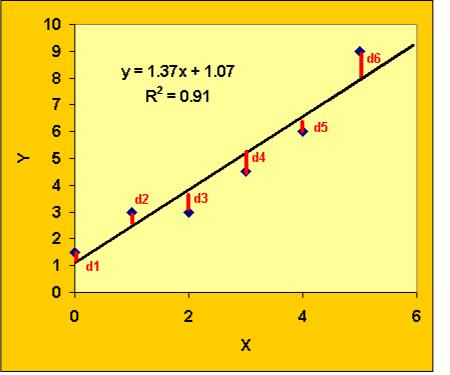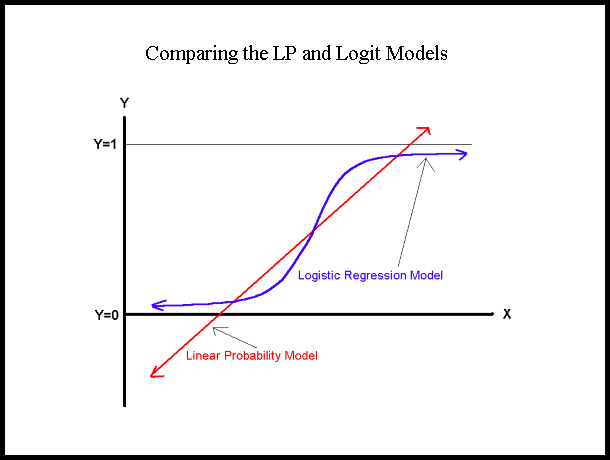# Difference between Linear and Logistic Regression

Linear and logistic regression are both forms of statistical analysis. That is the main reason why people often get confused between these two terms and interchange them. Both Linear and Logistic Regression represent a particular form of analysis that uses a different type or number of variables in statistics.

Undoubtedly, linear regression is the most basic and easy form of regression analysis. Here, the relationship between these two variables is assumed to be linear while analysis is used to find out the relation between responsive variables and exploratory variables. For instance, in linear regression, we can perform analysis on the price of a product and the quantity in which it is consumed. For this, we can use a random sample to perform our analysis. With the help of these results, regression analysis tends to find out the mathematical model which properly suits the data.

On the other hand, logistic regression is used for multiple variables, specifically for more than two variables. A logistic regression will form the mathematical model on the basis of available information and would start categorising the data and information. No doubt both forms of statistical analysis are used for forecasting and predicting in which linear regression is used for two variables while logistic regression is used for multiple variables. In addition, logistic regression is similar to multivariate variables. Linear regression is used for analysing quantitative variables and the result is also quantitative in nature. Similarly, the analysis done through logistic regression can be categorical or quantitative in nature.

Most importantly, if a regression is a linear function then the analysis will be linear regression but if the function is not linear it will be a non-linear regression.

### Instructions

• 1

Linear Regression:

Linear Regression is considered to be a basic form of statistical analysis. It is helpful in finding the relationships between two variables having a linear function between them.

- Image Courtesy: serc.carleton.edu• 2

Logistic Regression:

A more sophisticated and complex form of statistical analysis is logistic regression. This logistic regression resembles the multivariate regression and is very useful in doing quantitative and categorical analysis. Logistic regression is considered an advanced form of analysis that helps in predicting or forecasting various results.

- Image Courtesy: appstate.edu•